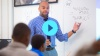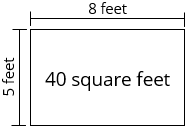# Area

Students develop an understanding of areas as how much two-dimensional space a figure takes up, and relate it to their work with multiplication from Units 2 and 3.

Math

Unit 4

## Unit Summary

In Unit 4, 3rd grade students understand area as how much two-dimensional space a figure takes up and relate it to their work with multiplication and division in Unit 2 and Unit 3

In early elementary grades, students may have informally compared area, seeing which of two figures takes up more space. In 2nd grade, students, partitioned a rectangle into rows and columns of same-sized squares and counted to find the total number of them, including using skip-counting and repeated addition to more efficiently do so (2.G.2, 2.OA.4).

At the beginning of this unit, students develop an understanding of area as an attribute of plane figures (3.MD.5) and measure it by counting unit squares (3.MD.6). After extensive work to develop students’ spatial structuring, students connect area to the operation of multiplication of length and width of the figure (3.MD.7a, b). Lastly, students connect the measure of area to both multiplication and addition, seeing with concrete cases that the area of a rectangle with whole-number side lengths $$a$$ and $$b + c$$ is the sum of $$a\times b$$ and $$a\times c$$ (3.MD.7c), and using the more general idea that area is additive to find the area of composite figures (3.MD.7d). The unit serves as a way to link topics and thinking across units, providing coherence between the work with multiplication and division in Units 2 and 3 (3.OA) with the work of area in this unit (3.MD.C).

Students will engage with many mathematical practices deeply in the unit. For example, students “use strategies for finding products and quotients that are based on the properties of operations; for example, to find [the area of a rectangle by multiplying] $$4\times 7$$, they may recognize that $$7 = 5 + 2$$ and compute $$4 \times 5 + 4 \times 2$$. This is an example of seeing and making use of structure (MP.7). Such reasoning processes amount to brief arguments that students may construct and critique (MP.3)” (PARCC Model Content Frameworks for Mathetmatics, p. 16). Further, students make use of physical tiles, rulers to relate side lengths to physical tiles, and later in the unit, the properties of operations themselves in order to find the area of a rectangle (MP.5). Additionally, “to build from spatial structuring to understanding the number of area-units as the product of number of units in a row and number of rows, students might draw rectangular arrays of squares and learn to determine the number of squares in each row with increasingly sophisticated strategies, such as skip-counting the number in each row and eventually multiplying the number in each row by the number of rows (MP.8)” (GM Progression, p. 17).

In Unit 5, students will study perimeter and distinguish between the measures of area and perimeter. Then, in future grades, students will rely on the understanding of area to solve increasingly complex problems involving area, perimeter, surface area, and volume (4.MD.3, 5.MD.3—5, 6.G.1—4). Students will also use this understanding outside of their study of geometry, as multi-digit multiplication problems in 4th grade (4.NBT.5), fraction multiplication in 5th grade (5.NF.4), and even polynomial multiplication problems in Algebra (A.APR.1) rely on an area model.

Pacing: 16 instructional days (14 lessons, 2 flex days, 1 assessment day)

Fishtank Plus for Math

Unlock features to optimize your prep time, plan engaging lessons, and monitor student progress.## Assessment

The following assessments accompany Unit 4.

### Pre-Unit

Have students complete the Pre-Unit Assessment and Pre-Unit Student Self-Assessment before starting the unit. Use the Pre-Unit Assessment Analysis Guide to identify gaps in foundational understanding and map out a plan for learning acceleration throughout the unit.

### Mid-Unit

Have students complete the Mid-Unit Assessment after lesson 9.

### Post-Unit

Use the resources below to assess student understanding of the unit content and action plan for future units.

Expanded Assessment Package

Use student data to drive your planning with an expanded suite of unit assessments to help gauge students’ facility with foundational skills and concepts, as well as their progress with unit content.

## Unit Prep

### Intellectual Prep

Unit Launch

Prepare to teach this unit by immersing yourself in the standards, big ideas, and connections to prior and future content. Unit Launches include a series of short videos, targeted readings, and opportunities for action planning.#### Intellectual Prep for All Units

• Read and annotate “Unit Summary” and “Essential Understandings” portion of the unit plan.
• Do all the Target Tasks and annotate them with the “Unit Summary” and “Essential Understandings” in mind.
• Take the Post-Unit Assessment.

#### Unit-Specific Intellectual Prep

 area model### Essential Understandings

• Area refers to the amount of space a two-dimensional figure takes up. One-dimensional figures have no volume.
• One can find the area of a rectangle by counting individual square units or by multiplying the length and width of the figure.
• Students “learn to rotate rectangular arrays physically and mentally, understanding that their areas are preserved under rotation, and thus, for example, $$4 \times 7 = 7 \times 4$$, illustrating the commutative property of multiplication” (Progressions for the Common Core State Standards in Mathematics, K-5, Geometric Measurement, p. 18).
• Students “learn to understand and explain that the area of a rectangular region of, for example, 12 length-units by 5 length-units can be found either by multiplying $$12\times5$$, or by adding two products, e.g., $$10\times5$$ and $$2\times5$$, illustrating the distributive property” (Progressions for the Common Core State Standards in Mathematics, K-5, Geometric Measurement, p. 18).
• One can “find areas of rectilinear figures by decomposing them into non-overlapping rectangles and adding the areas of the non-overlapping parts” (CCSS, standard 3.MD.7d). This is possible because area is additive.

### Vocabulary

area

length and width

square unit

unit square

To see all the vocabulary for Unit 4, view our 3rd Grade Vocabulary Glossary.

## Unit Practice

Word Problems and Fluency Activities

Access daily word problem practice and our content-aligned fluency activities created to help students strengthen their application and fluency skills.## Lesson Map

Topic A: Understanding Concepts of Area

Topic B: The Distributive Property and Composite Area

## Common Core Standards

Key

Major Cluster

Supporting Cluster

### Core Standards

#### Measurement and Data

• 3.MD.C.5 — Recognize area as an attribute of plane figures and understand concepts of area measurement.
• 3.MD.C.5.A — A square with side length 1 unit, called "a unit square," is said to have "one square unit" of area, and can be used to measure area.
• 3.MD.C.5.B — A plane figure which can be covered without gaps or overlaps by n unit squares is said to have an area of n square units.
• 3.MD.C.6 — Measure areas by counting unit squares (square cm, square m, square in, square ft, and improvised units).
• 3.MD.C.7 — Relate area to the operations of multiplication and addition.
• 3.MD.C.7.A — Find the area of a rectangle with whole-number side lengths by tiling it, and show that the area is the same as would be found by multiplying the side lengths.
• 3.MD.C.7.B — Multiply side lengths to find areas of rectangles with whole-number side lengths in the context of solving real world and mathematical problems, and represent whole-number products as rectangular areas in mathematical reasoning.
• 3.MD.C.7.C — Use tiling to show in a concrete case that the area of a rectangle with whole-number side lengths a and b + c is the sum of a × b and a × c. Use area models to represent the distributive property in mathematical reasoning.
• 3.MD.C.7.D — Recognize area as additive. Find areas of rectilinear figures by decomposing them into non-overlapping rectangles and adding the areas of the non-overlapping parts, applying this technique to solve real world problems.

• 1.G.A.2
• 2.G.A.2

• 2.MD.A.1

• 3.OA.B.5
• 3.OA.C.7

• 3.MD.D.8
• 4.MD.A.3
• 5.MD.C.3

• 5.NF.B.4

### Standards for Mathematical Practice

• CCSS.MATH.PRACTICE.MP1 — Make sense of problems and persevere in solving them.

• CCSS.MATH.PRACTICE.MP2 — Reason abstractly and quantitatively.

• CCSS.MATH.PRACTICE.MP3 — Construct viable arguments and critique the reasoning of others.

• CCSS.MATH.PRACTICE.MP4 — Model with mathematics.

• CCSS.MATH.PRACTICE.MP5 — Use appropriate tools strategically.

• CCSS.MATH.PRACTICE.MP6 — Attend to precision.

• CCSS.MATH.PRACTICE.MP7 — Look for and make use of structure.

• CCSS.MATH.PRACTICE.MP8 — Look for and express regularity in repeated reasoning.

Unit 3

Multiplication and Division, Part 2

Unit 5

Shapes and Their Perimeter

## Request a Demo

See all of the features of Fishtank in action and begin the conversation about adoption.

Yes

No

### Any other information you would like to provide about your school?